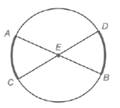Chapter 6.1, Problem 30EElementary Geometry For College St...

7th Edition
Alexander + 2 others
ISBN: 9781337614085

Solutions

Chapter
SectionElementary Geometry For College St...

7th Edition
Alexander + 2 others
ISBN: 9781337614085
Textbook Problem

In Exercises 30 and 31, complete each proof. Given: Diameters A B ¯ and C D ¯ in ⊙ E Prove: A C ⌢ ≅ D B ⌢PROOF Statements Reasons 1. ? 1. Given 2. ∠ A E C ≅ ∠ D E B 2. ? 3. m ∠ A E C = m ∠ D E B 3. ? 4. m ∠ A E C = m A C ⌢ and m ∠ D E B = m D B ⌢ 4. ? 5. m A C ⌢ = m D B ⌢ 5. ? 6. ? 6. If two arcs of a circle have the same measure, they are ≅

To determine

To prove: The ACDB by using the provided figure.

Explanation

Given: The diameters AB¯ and CD¯ in E

Postulate used:

Central angle postulate:

In a circle, the degree of a central angle is equal to the degree measure of its intercepted arc.

Definition:

In a circle or congruent circles, congruent arcs are arcs with equal measures.

Proof:

Step 1:

We need prove that if AB¯ and CD¯ are diameters of circle E, then ACDB.

 PROOF Statements Reasons 1. AB¯ and CD¯ are diameters of circle E 1. Given

Step 2:

The diameters AB¯ and CD¯ are form the vertical angles, AEC and DEB.

Since, vertical angles are congruent.

Therefore, the angles are congruent, then AEC and DEB have equal measures.

 PROOF Statements Reasons 1. AB¯ and CD¯ are diameters of circle E 1. Given 2. ∠AEC≅∠DEB 2. vertical angles are congruent. 3. m∠AEC=m∠DEB 3. congruent angles have equal measures.

Step 3:

Since, AEC and DEB are central angles.

Therefore, the measures degree of a central angle is equal to the degree measure of its intercepted arc.

Thus, mAC is the intercept arc of AEC and mDB is the intercept arc of DEB

Still sussing out bartleby?

Check out a sample textbook solution.

See a sample solution

The Solution to Your Study Problems

Bartleby provides explanations to thousands of textbook problems written by our experts, many with advanced degrees!

Get Started

Evaluate the integral. 26. 12w2lnwdw

Single Variable Calculus: Early Transcendentals

In Exercises 21-26, use the laws of logic to prove the propositions. [p(qq)(pq)]p(pq)

Finite Mathematics for the Managerial, Life, and Social Sciences

limx5x225x29x+20= a) 1 b) 10 c) d) does not exist

Study Guide for Stewart's Single Variable Calculus: Early Transcendentals, 8th